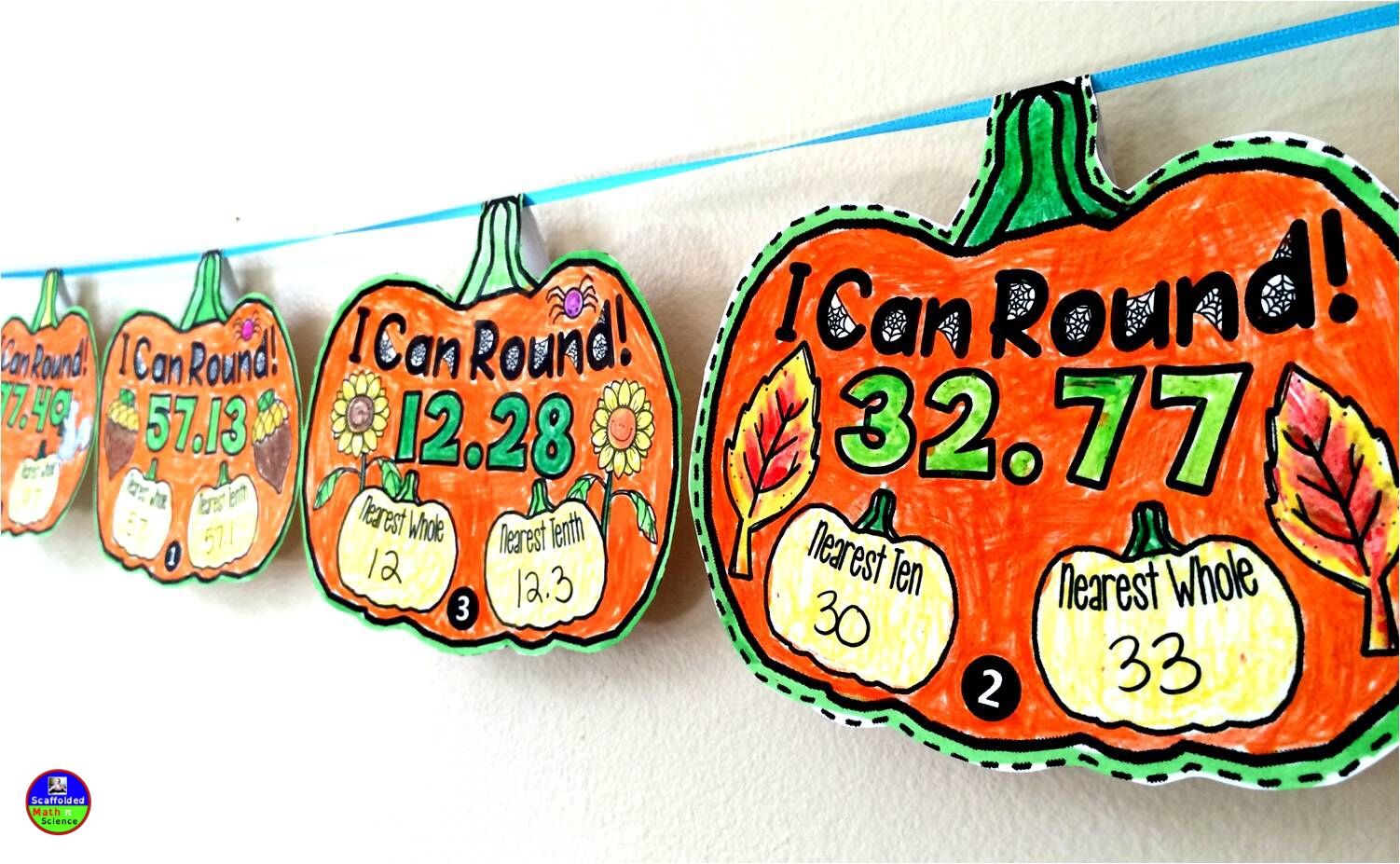# [小技巧]你真的了解C#中的Math.Round么？var num = Math.Round(12.125, 2);


# 四舍六入五成双

• 如果5前为奇数，就舍五进一
• 如果5前为偶数，就舍五不进（0是偶数）

1.15+1.25+1.35+1.45 = 5.2

1.2 + 1.3 + 1.4 + 1.5 = 5.4

1.2 + 1.2 + 1.4 + 1.4 = 5.2

# Math.Round的四舍五入

public static double Round (double value, int digits, MidpointRounding mode);
public static decimal Round (decimal d, int decimals, MidpointRounding mode);


• AwayFromZero - 四舍五入
• ToEven - 四舍六入五成双

var num = Math.Round(12.125, 2, MidpointRounding.AwayFromZero);

posted @ 2019-12-19 23:09  LamondLu  阅读(827)  评论(4编辑  收藏